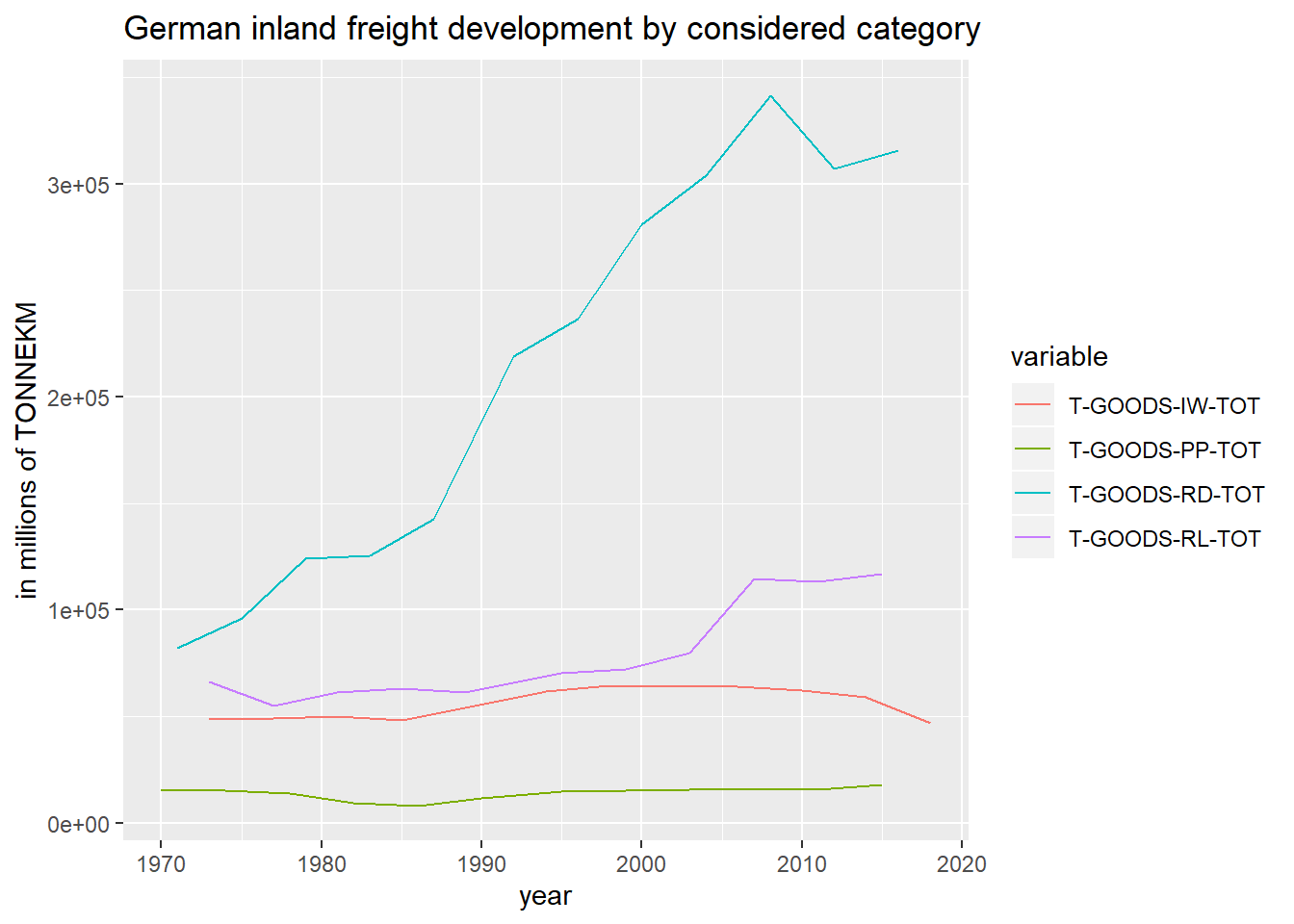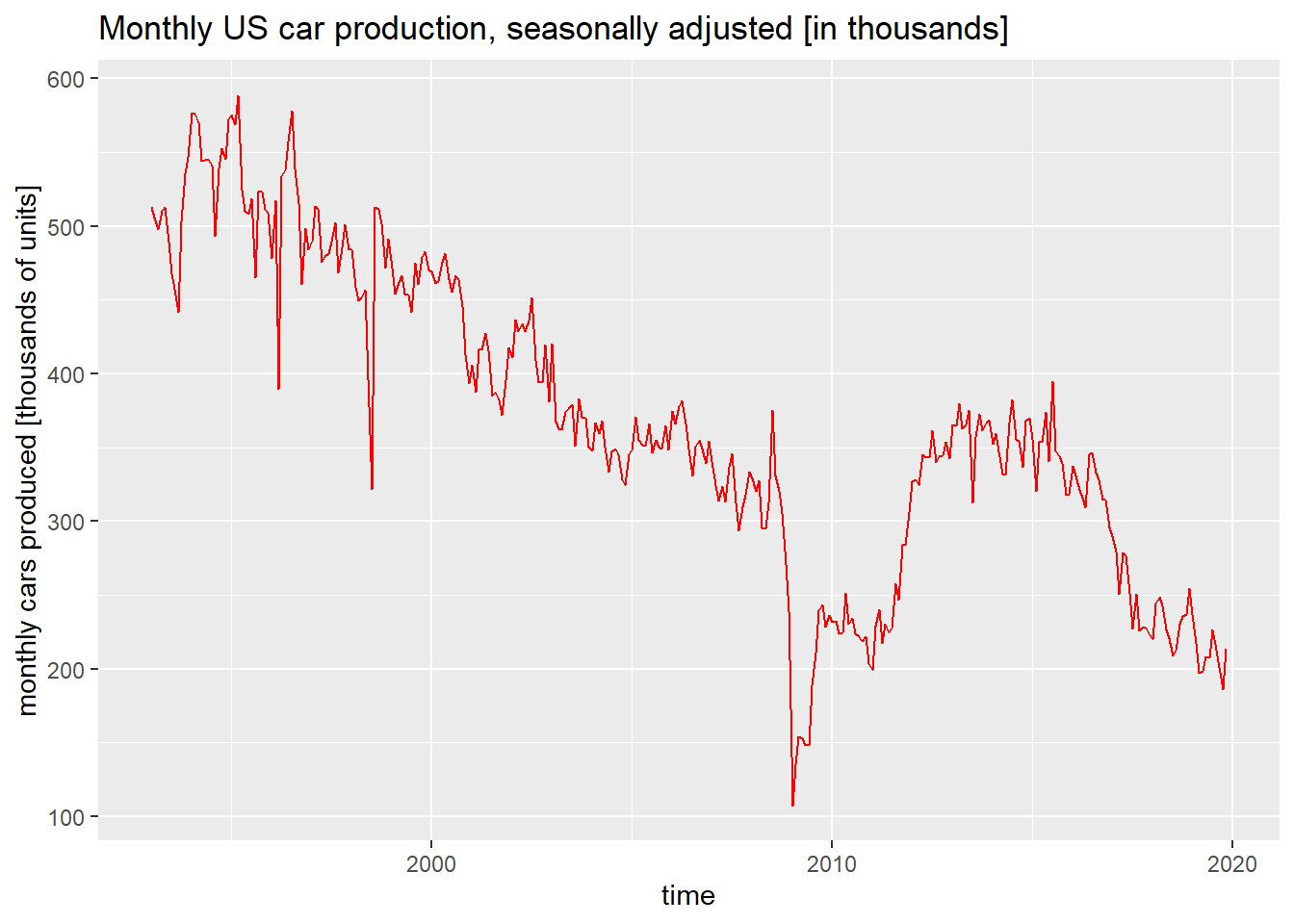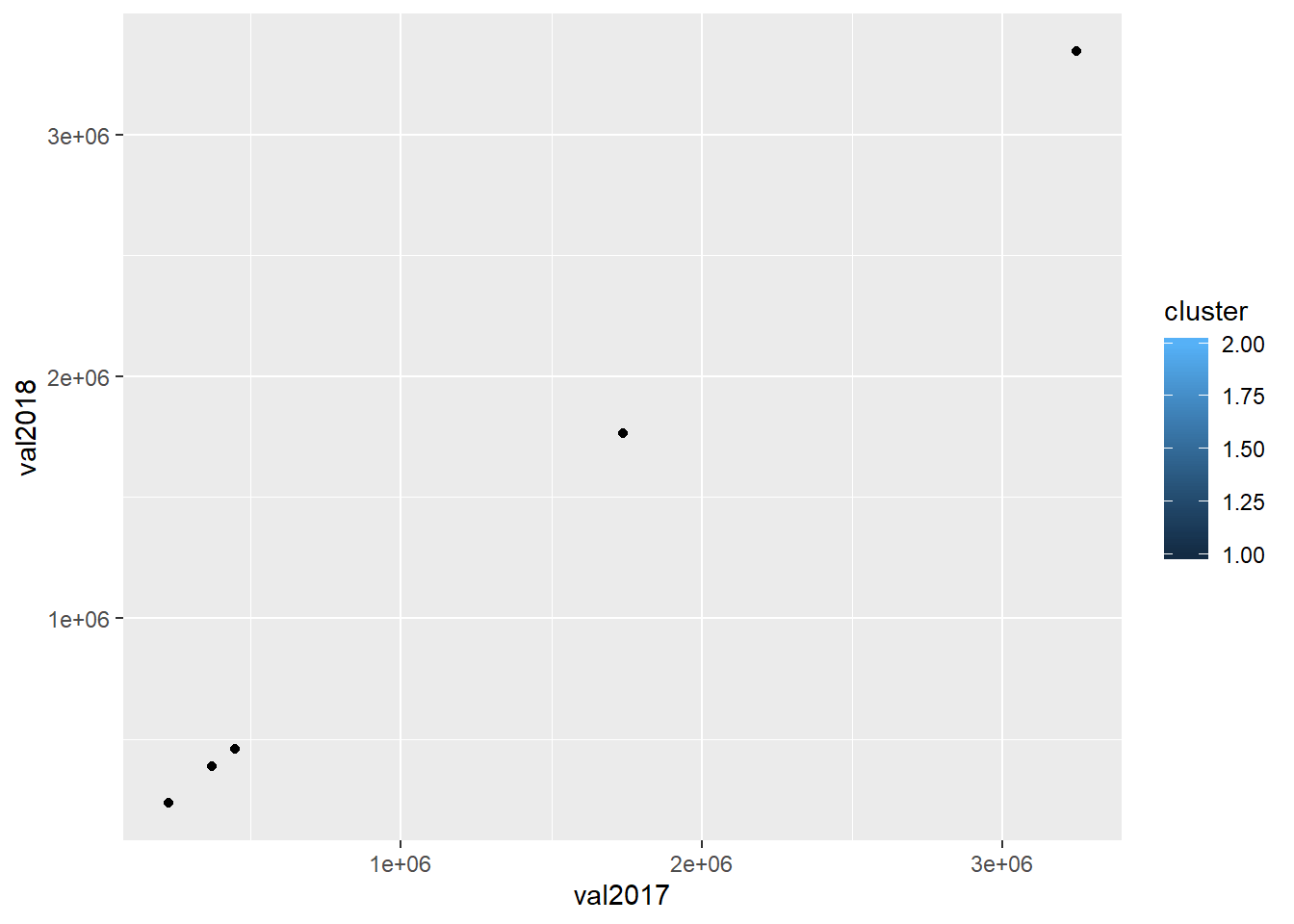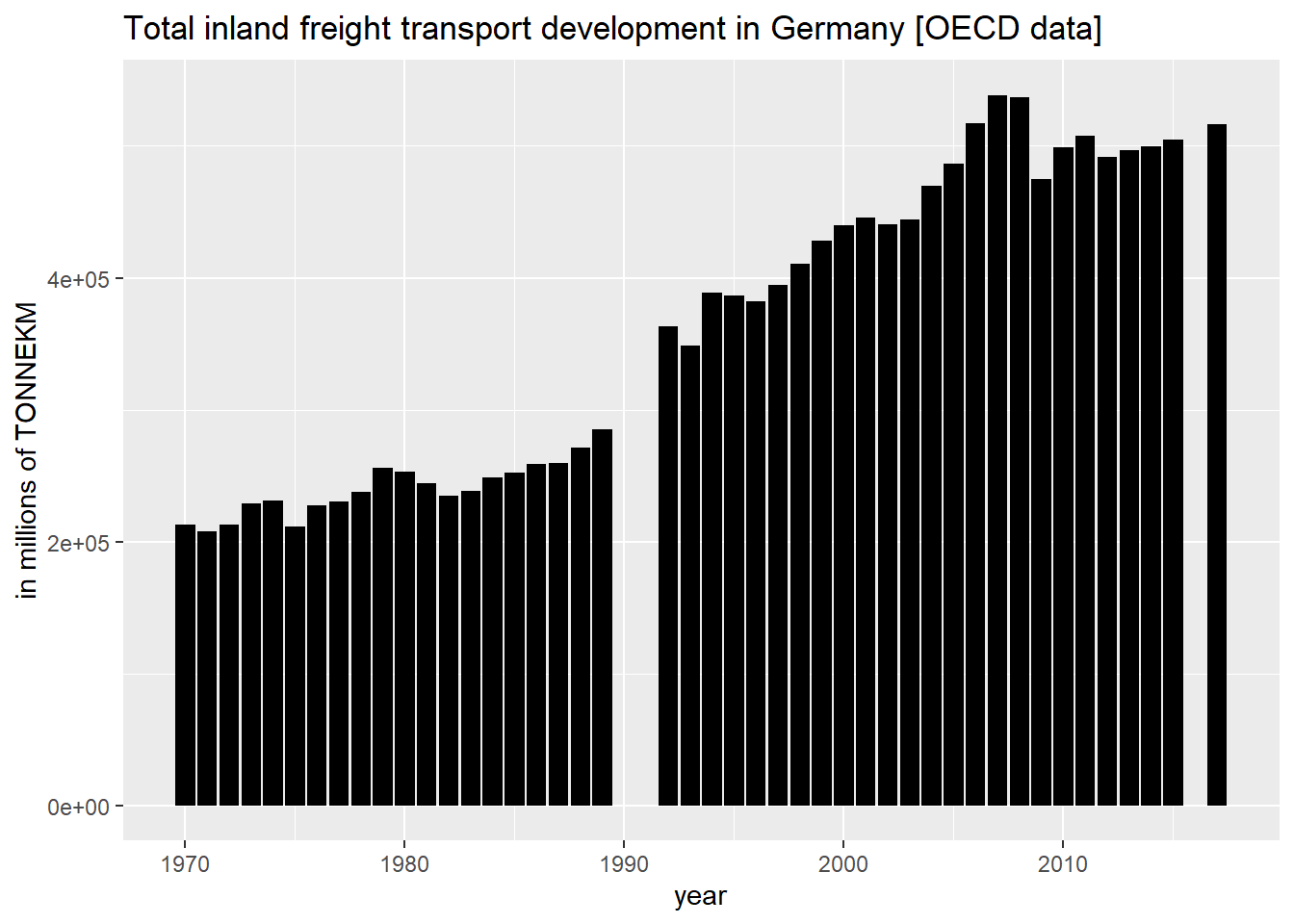# R programming

## Solving linear problem with fuzzy constraints by sampling beta with FuzzyLP in RIn a previous post I demonstrated how to use crispLP to solve a simple linear optimization problem with well-defined objective function and constraints. I have also demonstrated how to solve a fuzzy linear optimization problem with uncertain constraints, i.e. fuzzy constraint […]

## Linear optimization with fuzzy constraints conducted in R with FuzzyLPUsing the FuzzyLP package in R I have demonstrated simple linear optimization of a well defined linear problem using the crispLP function in a previous post. In that example basic simplex algorithm was applied to solve the problem. In this […]

## Simple linear problem with FuzzyLP, using crispLP and basic simplexIn previous posts I have demonstrated how to solve linear programs in R using lpSolve – or in Python using SciPy.optimize. In this coding example I want to show how to conduct simple linear optimization using the FuzzyLP package. FuzzyLP […]

## Comparing different German transport sectors by volume, using OECD data in RBased on my recent OECD-package related post (http://www.supplychaindataanalytics.com/oecd-package-interface-in-r-reading-german-freight-transport-data-from-oecd-directly-in-r/) I extend my recenet analysis of German transport volume development by comparing different inland freight categories in a ggplot-chart. The data, again, i querried using the OECD-package in R. From the previous […]

## Applying fredr package in R: Analyzing FRED domestic car production data for USAIn previous posts I showed how to retrieve and use data from Twitter (twitteR), Yahoo Finance (quantmod), The Guardian (guardianR) and OECD (oecd R-package). In this post I show how to access FRED data with an API key, using the […]

## K-Means clustering performed on OECD data in RA small example demonstrating how to use dplyr in R for filtering retrieved OECD data (pulled via R interface using the OECD R-package)

## OECD GDP data analysis in RAnalyzing GDP data with OECD package in Rm using German GDP development measured in EUR as example

## OECD package (interface) in R: Reading (German) freight transport data from OECD directly in R“OECD” is a package in R, providing an interface for performing a querry directly from R code. In this post I use the “OECD” package to analyze European transport data. I begin by reading in the OECD package in R. […]

## Gradient descent in R, for non-linear optimization (nloptr package)For solving transport problems or network modelling problems, linear programming will suffice. Nevertheless, depending on the topic at hand, non-linear programming might become relevant when considering additional constraints or objectives that are non-linear. R provides a package for solving non-linear […]